# WA Undecimated Wavelet Transform VI

LabVIEW 2014 Advanced Signal Processing Toolkit Help

Edition Date: June 2014

Part Number: 372656C-01

»View Product InfoDownload Help (Windows Only)

Owning Palette: Discrete Wavelet VIs

Computes the multi-level undecimated wavelet transform (UWT) of signal. This VI returns the approximation coefficients at the largest level and the detail coefficients at all levels for a 1D signal input and returns the approximation coefficients and the detail coefficients at all levels for a 2D signal input. The approximation coefficients and the detail coefficients at all levels are the same size as signal. The results of the UWT have the translation invariant property, which is helpful in robust feature extraction and pattern recognition. Wire data to the signal input to determine the polymorphic instance to use or manually select the instance.

Use the pull-down menu to select an instance of this VI.

 Select an instance WA 1D Undecimated Wavelet Transform (Waveform)WA 1D Undecimated Wavelet Transform (Array)WA 2D Undecimated Wavelet Transform

## WA 1D Undecimated Wavelet Transform (Waveform)signal specifies the input signal.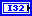levels specifies the number of levels in the discrete wavelet analysis. levels must be a positive integer no greater than log2(Ls), where Ls is the length of the 1D signal or the minimum dimensional size of the 2D signal. Otherwise, you can set the value to –1, which indicates that this VI sets levels as the largest integer no greater than log2(Ls). The default is –1.wavelet specifies the wavelet type to use for the discrete wavelet analysis. The default is db02. The options include two types: orthogonal (Haar, Daubechies (dbxx), Coiflets (coifx), Symmlets (symx)) and biorthogonal (Biorthogonal (biorx_x), including FBI (bior4_4 (FBI))), where x indicates the order of the wavelet. The higher the order, the smoother the wavelet. The orthogonal wavelets are not redundant and are suitable for signal or image denoising and compression. The biorthogonal wavelets usually have the linear phase property and are suitable for signal or image feature extraction. If you want to use other types of wavelets, do not wire this input. Instead, use the Wavelet Design Express VI to design the wavelet you want and wire the resulting analysis filters to the analysis filters input.error in describes error conditions that occur before this node runs. This input provides standard error in functionality.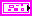analysis filters specifies the coefficients of the lowpass analysis filters and the highpass analysis filters for the wavelet you specify. If you specify a value for analysis filters, this VI ignores the settings in the wavelet input. You can use the Wavelet Design Express VI to design the analysis filters and the corresponding synthesis filters.lowpass specifies the coefficients of the lowpass analysis filter, which this VI uses to compute the approximation coefficients.highpass specifies the coefficients of the highpass analysis filter, which this VI uses to compute the detail coefficients.UWT coef returns the approximation coefficients and the detail coefficients from the multi-level undecimated wavelet transform (UWT) concatenated into an array of waveforms starting with the approximation coefficients at the largest level followed by the detail coefficients at all levels in descending order. You can use the WA Get Coefficients of Undecimated Wavelet Transform VI to read the approximation coefficients or detail coefficients at a specific level. Wire UWT coef to the UWT coef input of the WA Get Coefficients of Undecimated Wavelet Transform VI.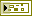error out contains error information. This output provides standard error out functionality.

## WA 1D Undecimated Wavelet Transform (Array)signal specifies the input signal.levels specifies the number of levels in the discrete wavelet analysis. levels must be a positive integer no greater than log2(Ls), where Ls is the length of the 1D signal or the minimum dimensional size of the 2D signal. Otherwise, you can set the value to –1, which indicates that this VI sets levels as the largest integer no greater than log2(Ls). The default is –1.wavelet specifies the wavelet type to use for the discrete wavelet analysis. The default is db02. The options include two types: orthogonal (Haar, Daubechies (dbxx), Coiflets (coifx), Symmlets (symx)) and biorthogonal (Biorthogonal (biorx_x), including FBI (bior4_4 (FBI))), where x indicates the order of the wavelet. The higher the order, the smoother the wavelet. The orthogonal wavelets are not redundant and are suitable for signal or image denoising and compression. The biorthogonal wavelets usually have the linear phase property and are suitable for signal or image feature extraction. If you want to use other types of wavelets, do not wire this input. Instead, use the Wavelet Design Express VI to design the wavelet you want and wire the resulting analysis filters to the analysis filters input.error in describes error conditions that occur before this node runs. This input provides standard error in functionality.analysis filters specifies the coefficients of the lowpass analysis filters and the highpass analysis filters for the wavelet you specify. If you specify a value for analysis filters, this VI ignores the settings in the wavelet input. You can use the Wavelet Design Express VI to design the analysis filters and the corresponding synthesis filters.lowpass specifies the coefficients of the lowpass analysis filter, which this VI uses to compute the approximation coefficients.highpass specifies the coefficients of the highpass analysis filter, which this VI uses to compute the detail coefficients.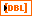UWT coef returns the approximation coefficients and the detail coefficients from the multi-level undecimated wavelet transform (UWT). The first row of UWT coef contains the approximation coefficients at the largest level. The other rows contain the detail coefficients at all levels in descending order. You can use the WA Get Coefficients of Undecimated Wavelet Transform VI to read the approximation coefficients or detail coefficients at a specific level. Wire UWT coef to the UWT coef input of the WA Get Coefficients of Undecimated Wavelet Transform VI.error out contains error information. This output provides standard error out functionality.

## WA 2D Undecimated Wavelet Transform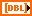signal specifies the 2D input signal.levels specifies the number of levels in the discrete wavelet analysis. levels must be a positive integer no greater than log2(Ls), where Ls is the length of the 1D signal or the minimum dimensional size of the 2D signal. Otherwise, you can set the value to –1, which indicates that this VI sets levels as the largest integer no greater than log2(Ls). The default is –1.wavelet specifies the wavelet type to use for the discrete wavelet analysis. The default is db02. The options include two types: orthogonal (Haar, Daubechies (dbxx), Coiflets (coifx), Symmlets (symx)) and biorthogonal (Biorthogonal (biorx_x), including FBI (bior4_4 (FBI))), where x indicates the order of the wavelet. The higher the order, the smoother the wavelet. The orthogonal wavelets are not redundant and are suitable for signal or image denoising and compression. The biorthogonal wavelets usually have the linear phase property and are suitable for signal or image feature extraction. If you want to use other types of wavelets, do not wire this input. Instead, use the Wavelet Design Express VI to design the wavelet you want and wire the resulting analysis filters to the analysis filters input.error in describes error conditions that occur before this node runs. This input provides standard error in functionality.analysis filters specifies the coefficients of the lowpass analysis filters and the highpass analysis filters for the wavelet you specify. If you specify a value for analysis filters, this VI ignores the settings in the wavelet input. You can use the Wavelet Design Express VI to design the analysis filters and the corresponding synthesis filters.lowpass specifies the coefficients of the lowpass analysis filter, which this VI uses to compute the approximation coefficients.highpass specifies the coefficients of the highpass analysis filter, which this VI uses to compute the detail coefficients.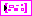UWT coef returns the approximation coefficients and the detail coefficients from the multi-level undecimated wavelet transform (UWT). Each element of the array contains the 2D UWT results of one level. The ith element stores the approximation coefficients and the detail coefficients at level i+1. You can use the WA Get Coefficients of Undecimated Wavelet Transform VI to read the approximation coefficients or detail coefficients at a specific level. Wire UWT coef to the UWT coef input of the WA Get Coefficients of Undecimated Wavelet Transform VI.low_low returns the approximation coefficients from the lowpass analysis filtering on each row and each column. The low_low coefficients are a low-resolution approximation of the original 2D signal.low_high returns the detail coefficients from the lowpass analysis filtering on each row and the highpass analysis filtering on each column. The high-frequency signal along the column direction influences the low_high coefficients.high_low returns the detail coefficients from the highpass analysis filtering on each row and the lowpass analysis filtering on each column. The high-frequency signal along the row direction influences the high_low coefficients.high_high returns the detail coefficients from the highpass analysis filtering on each row and each column. The high-frequency signal along the diagonal direction influences the high_high coefficients.error out contains error information. This output provides standard error out functionality.

## WA Undecimated Wavelet Transform Details

The following illustration shows an example of a three-level UWT, where you set the levels input of this VI to 3. The length of the input signal is 16 points.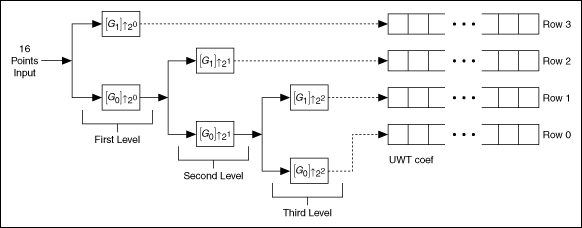where the subscript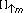indicates the up-sampling by a factor of m. Using information in the previous illustration, you can see that the UWT coef output contains the approximation coefficients of the largest level and the detail coefficients of each level.

In addition, compared to the discrete wavelet transform (DWT), the UWT does not have sub-sampling. Therefore, the output of each level has the same length as the input signal.

Undecimated discrete wavelet transform is translation-invariant. In other words, when the input signal shifts with certain taps, the output coefficients shift by the same taps, which is important for robust feature extractions, such as peak detection.